Figures for "Truchet curves and surfaces"

Cameron Browne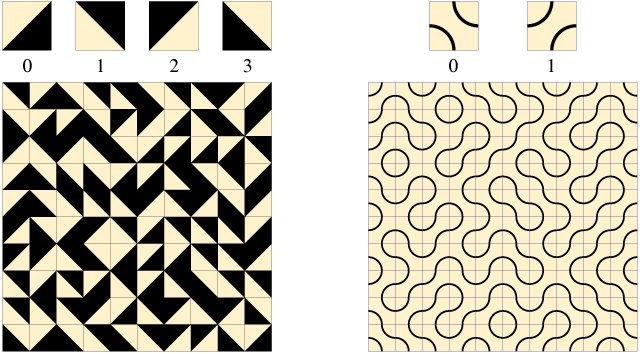Figure 1. Truchet's original tiles (left) and their popular incarnation (right).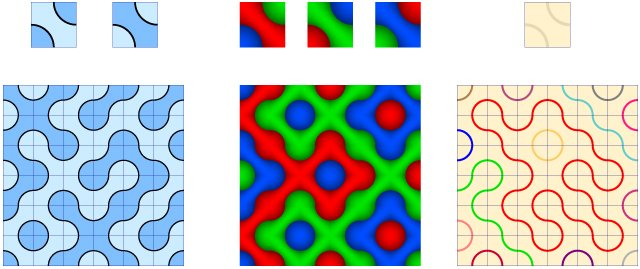Figure 2. Different styles: duotone, tritone and path colouring.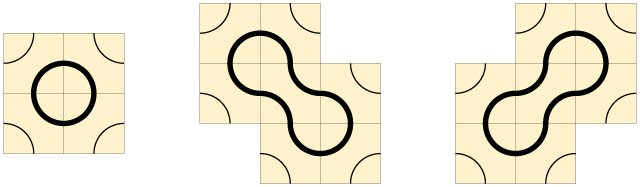Figure 3. The simplest truchet contours: circle, left dumbbell and right dumbbell.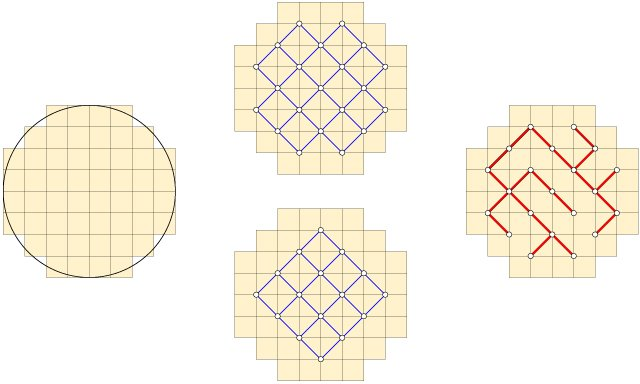Figure 4. Grid of cells filling a source shape (circle), two corresponding graphs and a spanning tree.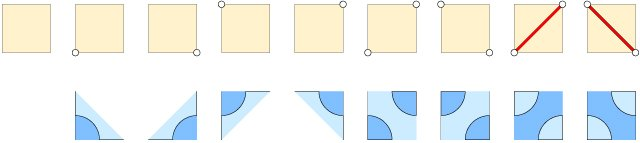Figure 5. Graph-to-contour replacement rules.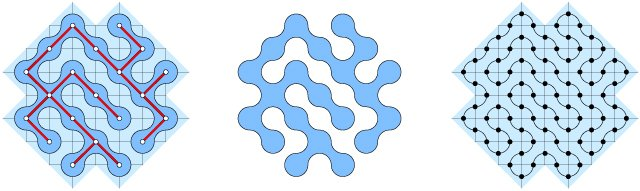Figure 6. Closed contour with graph overlaid, the final shape and the hamiltonian cycle within its footprint.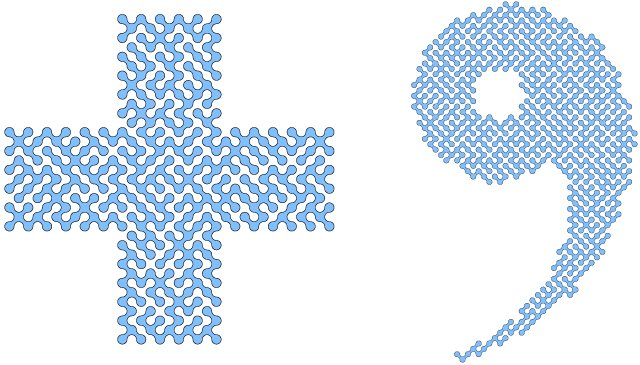Figure 7. More detailed ST contours.Figure 8. Cutting edges to determine perimeter length (left) and basic area considerations (right).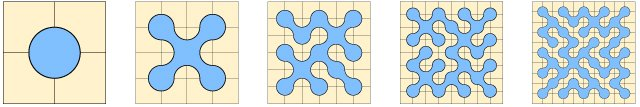Figure 9. ST contours for even-sided grids 2x2, 4x4, 6x6, 8x8 and 10x10.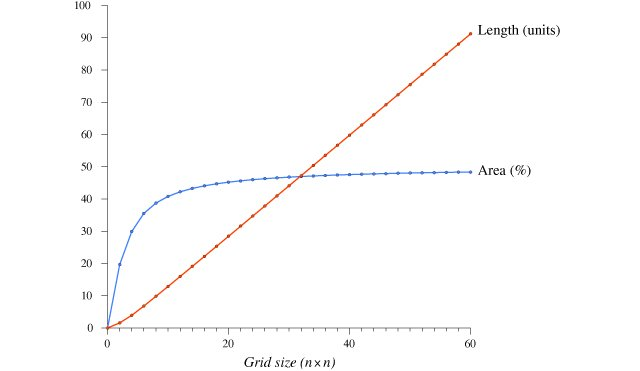Figure 10. Graph of contour perimeter and area for even-sided grids up to 60x60.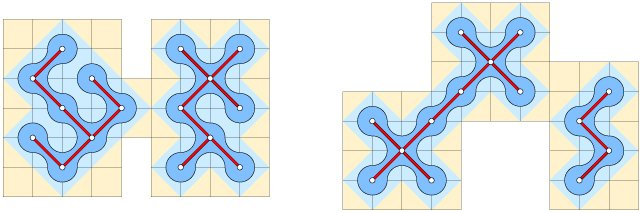Figure 11. Impassable bottlenecks split the contour.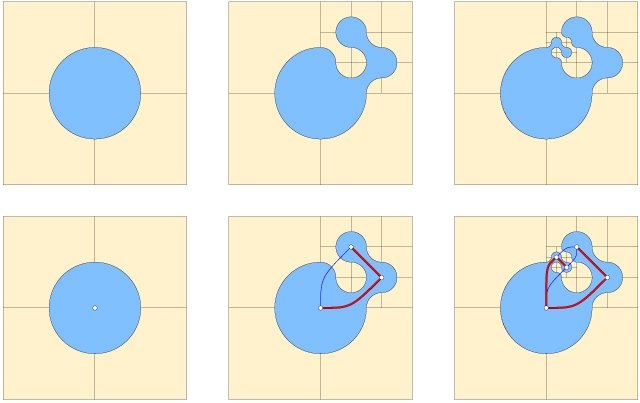Figure 12. Multiresolution ST contours (top row) and their corresponding graphs (bottom row).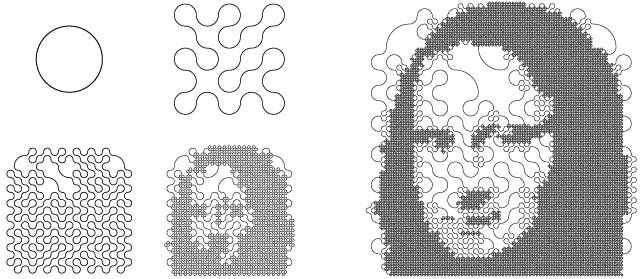Figure 13. Halftoning by multiresolution ST contour. Each step consists of a single curve.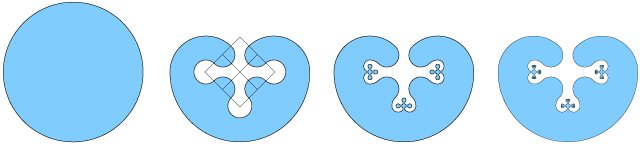Figure 14. Three iterations of the kidney fractal.Figure 15. Three-dimensional truchet pipes.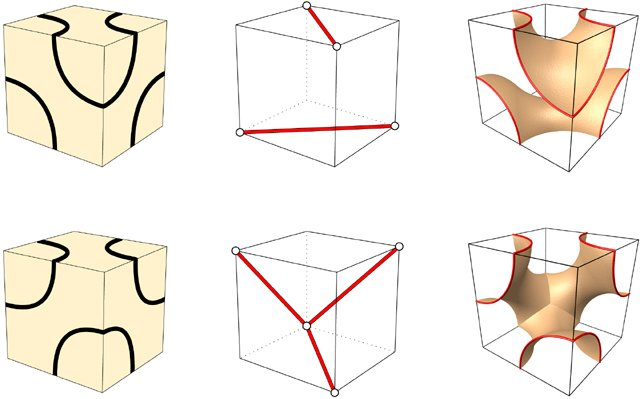Figure 16. Two truchet-tiled cubes, their underlying graphs and the resulting surface patches.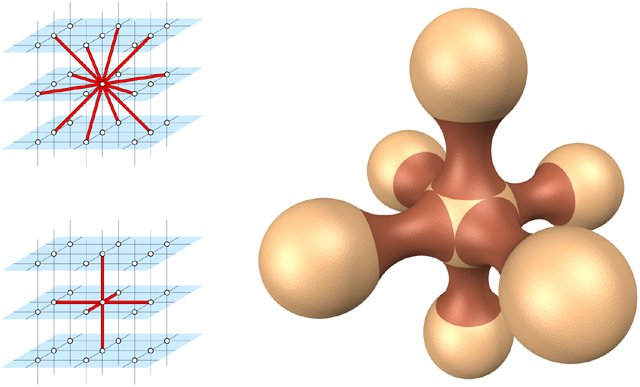Figure 17. Diagonal and orthogonal lattice adjacencies (left) and an orthogonally based truchet surface (right).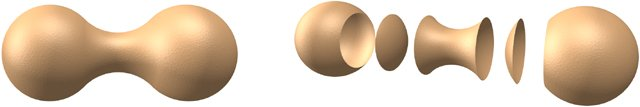Figure 18. A three-dimensional dumbbell and its component parts: 2 spheres + 1 neck - 2 caps.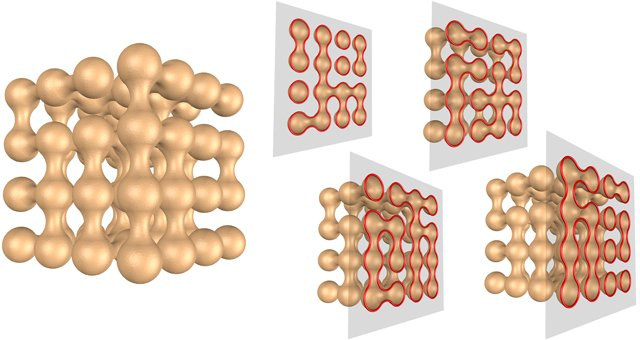Figure 19. Axial slices through the surface reveal truchet contours at each unit interval.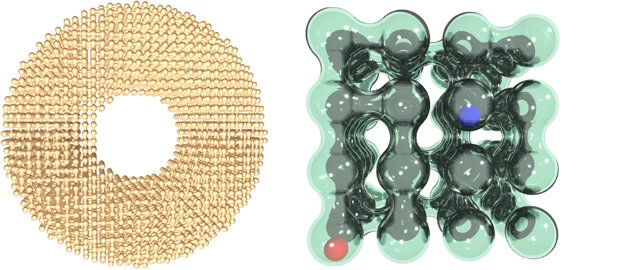Figure 20. A truchet surface filling a torus with 6,120 vertices and a truchet glass bead puzzle.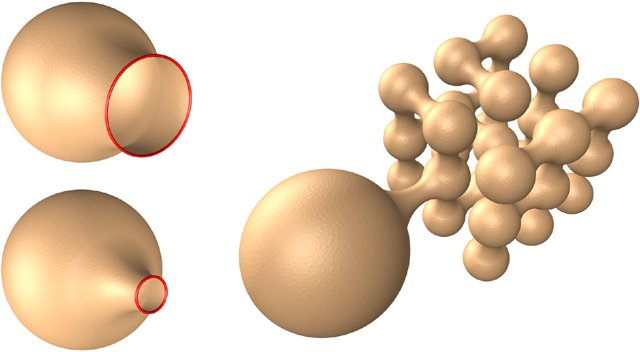Figure 21. Multiresolution surface development.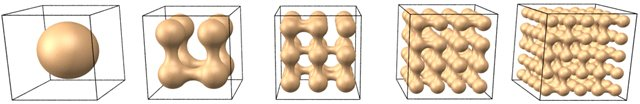Figure 22. Truchet surfaces fitted to cubic lattices 2x2x2, 3x3x3, 4x4x4, 5x5x5 and 6x6x6.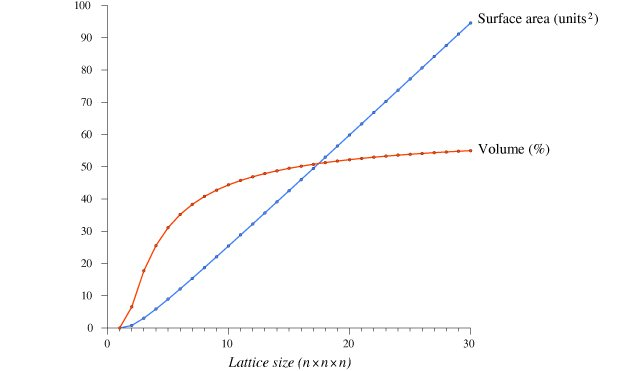Figure 23. Graph of surface area and volume for cubic lattices up to 30x30x30.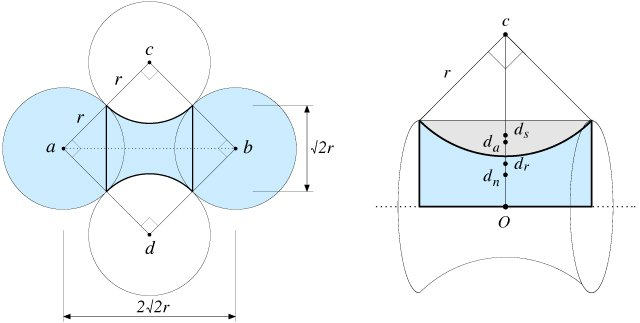Figure 24. Neck geometry (left) and neck construction as a surface of revolution (right).